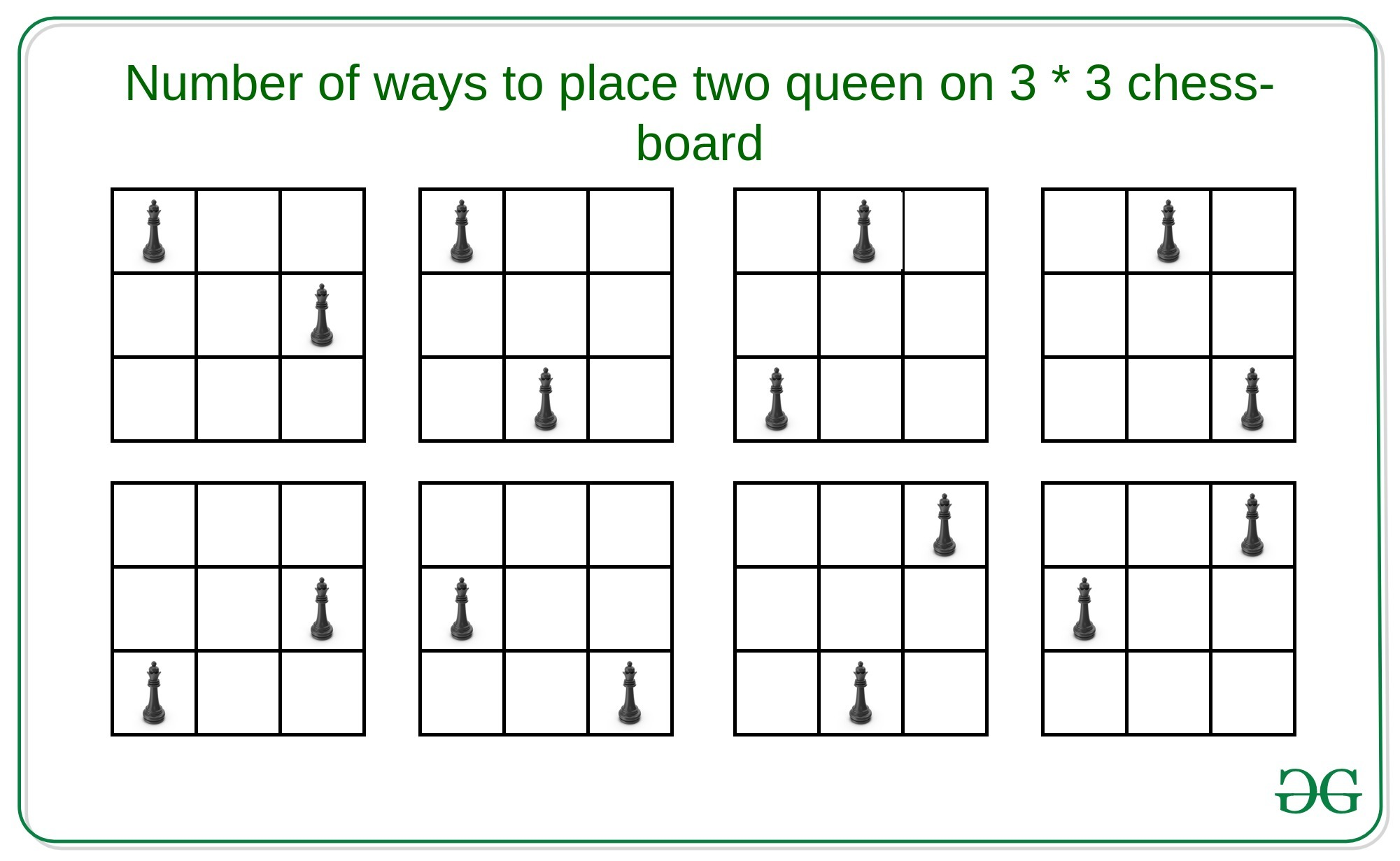# Number of ways to place two queens on a N*N chess-board

• Last Updated : 20 Apr, 2021

Given an integer N denoting a N * N chess-board, the task is to count the number of ways to place two queens on the board such that, they do no attack each other.
Examples:

Input: N = 9
Output: 2184
Explanation:
There are 2184 ways to place two queens on 9 * 9 chess-board.
Input: N = 3
Output:
Explanation:
There are 8 ways to place two queens on 3 * 3 chess-board.

Attention reader! Don’t stop learning now. Get hold of all the important DSA concepts with the DSA Self Paced Course at a student-friendly price and become industry ready.  To complete your preparation from learning a language to DS Algo and many more,  please refer Complete Interview Preparation Course.

In case you wish to attend live classes with experts, please refer DSA Live Classes for Working Professionals and Competitive Programming Live for Students.Naive Approach: A simple solution will be to choose two every possible position for the two queens on the N * N matrix and check that they are not in horizontal, vertical, positive diagonal or negative diagonal. If yes then increment the count by 1.
Time Complexity: O(N4)
Efficient Approach: The idea is to use combinations to compute the possible positions of the queens such that they do not attack each other. A useful observation is that it is quite easy to calculate the number of positions that a single queen attacks. That is –

```Number of positions a queen attack =
(N - 1) + (N - 1) + (D - 1)

Here,
// First N-1 denotes positions in horizontal direction
// Second N-1 denotes positions in vertical direction
// D = Number of positions in
positive and negative diagonal```

If we do not place the queen on the last row and the last column then the answer will simply be the number of positions to place in a chessboard of (N-1)*(N-1), whereas if we place in the last column and last row then possible positions for queens will be 2*N – 1 and attacking at 3*(N – 1) positions. Therefore, the possible positions for the other queen will be N2 – 3*(N-1) – 1. Finally, there are (N-1)*(N-2) combinations where both queens are on the last row and last column. Therefore, the recurrence relation will be –

```Q(N) = Q(N-1) + (N2-3*(N-1)-1)-(N-1)*(N-2)

// By Induction
Q(N) = (N4)/2 - 5*(N3)/3 + 3*(N2)/2 - N/3```

Below is the implementation of the above approach:

## C++

 `// C++ implementation to find the` `// number of ways to place two ` `// queens on the N * N chess board`   `#include `   `#define ll long long` `using` `namespace` `std;`   `// Function to find number of valid ` `// positions for two queens in the` `// N * N chess board` `ll possiblePositions(ll n)` `{` `    ``ll term1 = ``pow``(n, 4);` `    ``ll term2 = ``pow``(n, 3);` `    ``ll term3 = ``pow``(n, 2);` `    ``ll term4 = n / 3;` `    ``ll ans = (``ceil``)(term1) / 2 - ` `             ``(``ceil``)(5 * term2) / 3 + ` `             ``(``ceil``)(3 * term3) / 2 - term4;` `    ``return` `ans;` `}`   `// Driver Code` `int` `main()` `{` `    ``ll n;` `    ``n = 3;` `    `  `    ``// Function Call` `    ``ll ans = possiblePositions(n);` `    ``cout << ans << endl;` `    ``return` `0;` `}`

## Java

 `// Java implementation to find the` `// number of ways to place two ` `// queens on the N * N chess board` `class` `GFG{`   `// Function to find number of valid ` `// positions for two queens in the` `// N * N chess board` `static` `double` `possiblePositions(``double` `n)` `{` `    ``double` `term1 = Math.pow(n, ``4``);` `    ``double` `term2 = Math.pow(n, ``3``);` `    ``double` `term3 = Math.pow(n, ``2``);` `    ``double` `term4 = n / ``3``;` `    ``double` `ans = (Math.ceil(term1 / ``2``)) - ` `                 ``(Math.ceil(``5` `* term2) / ``3``) + ` `                 ``(Math.ceil(``3` `* term3) / ``2``) - term4;`   `    ``return` `(``long``)ans;` `}`   `// Driver Code` `public` `static` `void` `main(String[] args)` `{` `    ``double` `n;` `    ``n = ``3``;` `    `  `    ``// Function Call` `    ``double` `ans = possiblePositions(n);` `    ``System.out.print(ans + ``"\n"``);` `}` `}`   `// This code is contributed by sapnasingh4991`

## Python3

 `# Python3 implementation to find the ` `# number of ways to place two ` `# queens on the N * N chess board ` `import` `math `   `# Function to find number of valid ` `# positions for two queens in the ` `# N * N chess board ` `def` `possiblePositions(n): ` `    `  `    ``term1 ``=` `pow``(n, ``4``); ` `    ``term2 ``=` `pow``(n, ``3``); ` `    ``term3 ``=` `pow``(n, ``2``); ` `    ``term4 ``=` `n ``/` `3``; ` `    `  `    ``ans ``=` `((math.ceil(term1)) ``/` `2` `-` `           ``(math.ceil(``5` `*` `term2)) ``/` `3` `+` `           ``(math.ceil(``3` `*` `term3)) ``/` `2` `-` `term4); ` `           `  `    ``return` `ans; `   `# Driver code ` `if` `__name__ ``=``=` `'__main__'``: ` `    `  `    ``n ``=` `3`   `    ``# Function call` `    ``ans ``=` `possiblePositions(n) ` `    `  `    ``print``(``int``(ans)) `   `# This code is contributed by jana_sayantan`

## C#

 `// C# implementation to find the` `// number of ways to place two ` `// queens on the N * N chess board` `using` `System;`   `class` `GFG{`   `// Function to find number of valid ` `// positions for two queens in the` `// N * N chess board` `static` `double` `possiblePositions(``double` `n)` `{` `    ``double` `term1 = Math.Pow(n, 4);` `    ``double` `term2 = Math.Pow(n, 3);` `    ``double` `term3 = Math.Pow(n, 2);` `    ``double` `term4 = n / 3;` `    ``double` `ans = (Math.Ceiling(term1 / 2)) - ` `                 ``(Math.Ceiling(5 * term2) / 3) + ` `                 ``(Math.Ceiling(3 * term3) / 2) - term4;`   `    ``return` `(``long``)ans;` `}`   `// Driver Code` `public` `static` `void` `Main(String[] args)` `{` `    ``double` `n;` `    ``n = 3;` `    `  `    ``// Function Call` `    ``double` `ans = possiblePositions(n);` `    ``Console.Write(ans + ``"\n"``);` `}` `}`   `// This code is contributed by Amit Katiyar`

## Javascript

 ``

Output:

`8`

Time Complexity: O(1)

My Personal Notes arrow_drop_up
Recommended Articles
Page :